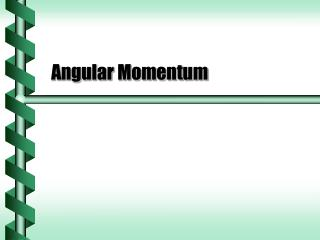DownloadDownload PresentationAngular Momentum

# Angular Momentum

Télécharger la présentation## Angular Momentum

- - - - - - - - - - - - - - - - - - - - - - - - - - - E N D - - - - - - - - - - - - - - - - - - - - - - - - - - -
##### Presentation Transcript

1. Angular Momentum

2. Inertia and Velocity • In the law of action we began with mass and acceleration • F = ma • This was generalized to use momentum: p = mv.

3. Moment of Momentum • To continue the analysis of rotational motion, we must also extend the idea of momentum. p r

4. Law of Angular Inertia • The time derivative of angular momentum is the net torque. • A net external torque causes a change in angular momentum.

5. Applying Torque • An external torque changes angular momentum. • dL/dt= t L L+rpsinq w w p

6. Spinning Mass • The moment of inertia is the analog of mass for rotational motion. • The analog for angular momentum would be: w

7. Conservation • With no external torque, angular momentum is constant. • dL/dt= 0, L = constant 4w w m r/2 r I = mr2 I = mr2/4 next